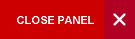## ECON1005: INTRODUCTION TO STATISTICS

This course is designed to teach students various concepts in descriptive and inferential statistics. It is also designed to give students an introduction to research methods.

Outline: Describing a data set (graphical methods, measures of central tendency, measures of variability, measures of shape); probability and probability distributions (sample space, probability of events, events composition, conditionality and independence, random variables, expectation for discrete random variables, binomial distribution, normal distribution), sampling distributions (central limit theorem), large-sample estimation (confidence intervals, choosing a sample size); large-sample tests of hypotheses.

Pre-requisites: CSEC/CXC Math or GCE Math or ECON0001 or GOVT0100 (Anti-requisite: SOCI1005 or STAT1001)

Semester: 1 & 2

Lecturer: Dr. Kelly-Ann Dixon-Hamil

Position: Lecturer

Office Hours:

Office Location: Department Of Economics Main Block, Room15